Objective :
To create a Breakout game using accelerators and materials of FPGA using Vivado and Nexys4 board.
Primary language: VHDL.

Connections :
We declare the entries and the exits in the "entity". Then, we make connections using "entity work._nomdel’entity_ (behavioral) port map" and declare the intermediate inputs and outputs as signals.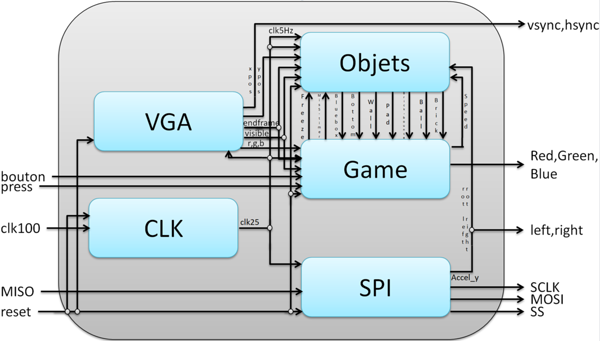Frequency Conversion :

To connect the Object entity we had to use a "clk5Hz". Hence, we made a conversion code of 25MHz to 5Hz using a counter code.
With the inversely proportional relation of frequency and period, it could be found that 5 x 10^6 periods of 25MHz = 1 period of 5Hz. So for one half of the periods of clk25 we give the value '1' and for the other half the value '0' at clk5Hz.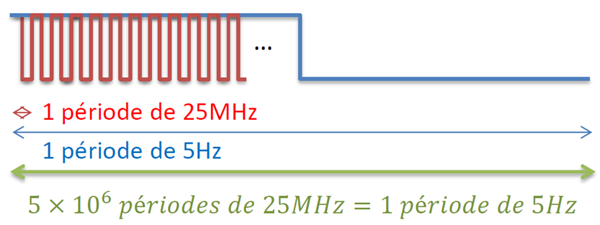Racket Movement :

For the racket movement, left i and right i, we have created a code that depends on an accelerometer motion signal which is the "acc (10)". We chose this one as it was less sensitive compared to the other signals when we tested them with an accelerometer code.
We have made an additional amelioration by increasing the sensibility of the accelerometer.

Score Display :
7 Segments Display :
To achieve this, it was necessary to understand the operation of anodes and cathodes of the board. The numbers from 0 to 9 are created using the 7 segments.
It was also important to understand the "Refresh Period" which is from 1ms to 16ms. This corresponds to the refresh rate of 62.5Hz at 1kHz.
We decided to recover a frequency of about 1kHz by converting the clk25.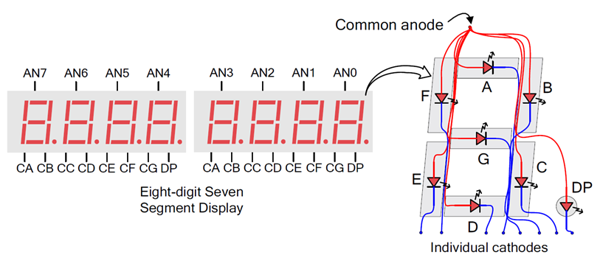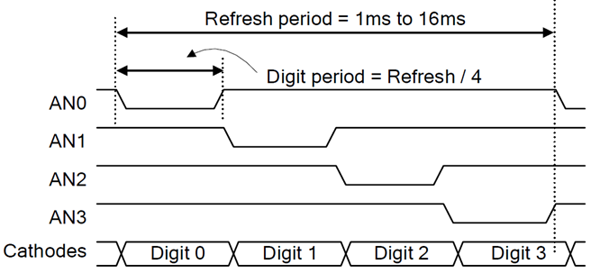Display of 2 independent digits :
This "Refresh Frequency" is required to display more than 2 independent digits at the same time. So with this code, at each rising edge of frequency, we have an infinite loop that chooses the first digit and the second digit one after the other.
For the Broken Brick Count Counter, a variable has been created in the brick_bounce file that gets incremented each time a brick is broken.
Thus, with the 2 mathematical equations, we recover the 1st digit and the 2nd digit of this number separately. (Numbers in base 10 -> Code BCD (Binary Coded Decimal))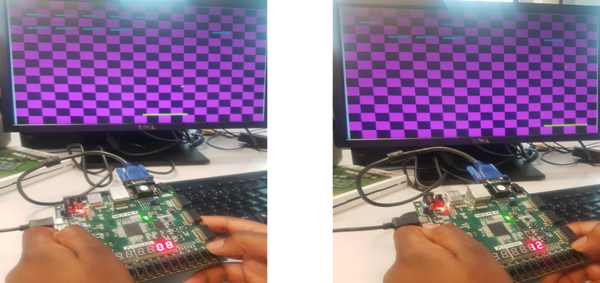Displaying a Message (in Pixels) : WIN or LOSE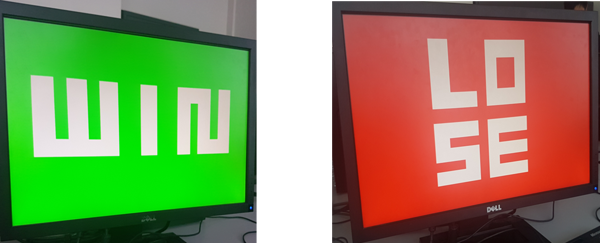Level Change :
• Ball Speed
• Color of the checkerboard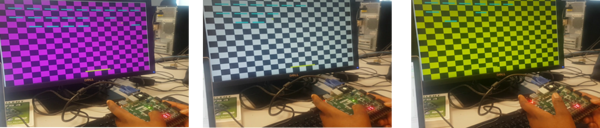Level 1         Level 2         Level 3

VHDL Implementation :